List of Notations in the Book
- Practical Electron Microscopy and Database -
- An Online Book -

https://www.globalsino.com/EM/

This book (Practical Electron Microscopy and Database) is a reference for TEM and SEM students, operators, engineers, technicians, managers, and researchers.

=================================================================================

Table 3713. List of notations in the book.

 Symbols Meanings Examples a Diameter of the objective aperture a A lattice or cell parameter: length of a basis vector, length of a cell edge a' A new basis vector after a transformation of the coordinate system a (or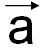) One of the basis vectors of direct lattice a* Length of a basis vector of reciprocal lattice a* A basis vector of reciprocal lattice A Thickness of the crystal in the TEM specimen page3769 A Atomic weight of the atoms in the specimen page2673 A(k) The aperture function describing the effect of the objective aperture page3732 A(u) Aperture function page4236 Ad Dimensionless atomic mass        . page2673 AS Surface area of a sphere page2671, page4631 b A lattice or cell parameter: length of a basis vector, length of a cell edge page3464 b (or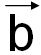) One of the basis vectors of direct lattice: Burgers vector b' A new basis vector after a transformation of the coordinate system b* Length of a basis vector of reciprocal lattice b* A basis vector of reciprocal lattice c (or) One of the basis vectors of direct lattice c A lattice or cell parameter: length of a basis vector, length of a cell edge c' A new basis vector after a transformation of the coordinate system c* Length of a basis vector of reciprocal lattice c* A basis vector of reciprocal lattice Cc Chromatic aberration coefficient page4165 Cn Rotation axis in crystallography (1, 2, 3, 4 and 6) page1622 Cs Spherical aberration of the objective lens page1715 C1s Excitation coefficient for plane wave incidence page4217 d Diameter of TEM specimen over which the illumination is coherent page1708 D(k) Envelope function page3879 dhkl Lattice-plane spacing of the (hkl) planes, i.e. interplanar distance of neighbouring net planes (hkl) e Elementary charge EL2,3 Si L2,3 energy loss onset of the silicon compounds page3441 E(u) Envelope functions, including Es and Ec page4236 Et(|g|) or Et(u) Temporal-coherence envelope function page4236, page4165 E0 Incident electron energy page4217 E1s The energy of the lowest energy bound state page4217 En (Euclidean) point space of dimension n ΔE Energy range of the PEEL spectra page3384 Es(|g|) Spatial-coherence envelope function page4236, page4153 f(θ) Atomic scattering factor page1421 fe(s) Atomic scattering factor for electrons F(g) or Fhkl Structure factor of the cell, corresponding to the Bragg reflection hkl fX Atomic scattering factor for x-rays F Fourier transform F-1 Inverse Fourier transform |F(hkl)|; or |F| Modulus of the structure factor F(hkl) g Spatial frequency page1715 |g| The spatial frequency page3732 gij Element of metric tensor ghkl Reciprocal-space vector for the planes with indices hkl G Metric tensor of direct lattice G* Metric tensor of reciprocal lattice h Planck’s constant h Reciprocal-lattice vector hkl Miller indices: indices of the Bragg reflection (Laue indices) from the set of parellel equidistant net planes (hkl) page2506 hkl Coordinates of a reciprocal-lattice point, coefficients of reciprocal-lattice vector r* (hkil) Bravais-Miller indices: indices of a crystal face, or of a single net plane, for the hexagonal axes a1, a2, a3, c (hkl) Miller indices: indices of a crystal face, or of a single net plane page2506 {hkl} Indices of a set of all symmetrically equivalent crystal faces or net planes, i.e., the family of all the planes that are equivalent to (hkl) by the symmetry of the lattice page2506 {hkil} Indices of a set of all symmetrically equivalent crsytal faces or net planes for the hexagonal axes a1, a2, a3, c [hkl] A direction that denotes the direction in the basis of the direct lattice vectors instead of the reciprocal lattice page2506 A family of the directions that are equivalent to [hkl] by symmetry page2506 i Center of inversion (center of symmetry) page1614 I0 Objective lens current page4165 I0 Intensity of the incident electron beam, page3769 k The reciprocal space vector page3732 L Vector lattice m Mirror planes in crystals page1620 m Relativistic electron mass m0 Electron rest mass page4787 mp Proton mass page2673 M Symmetry operation or motion n Dimension of a space N Atomic density page2673 NA Avogadro’s number page4475, page2673 p(r) Point spread function of a system page4907 Puvw Probability of seeing a family of zone axes P(xyz) Patterson function at the point x,y,z qe(r) Specimen transmission function Q Probability of scattering an electron page2673Scattering vector page1421 r Length of the position vector r (or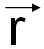) r (or) Position vector of a point or an atom: The real space coordinates, three-dimensional position vector in direct space page3732 r* Reciprocal-lattice vector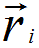Position of atom i page1421 s |g|/2 S Illuminating area by the incident electron beam page3769 S Point spread function (PSF) of a system page2621 t Length of a translation vector t t Translation vector t(r) Spread function of the objective lens T(u) Microscope transfer function u Spatial frequency page3699 u The reciprocal space coordinates page3732 u Two-dimensional vector in the back focal plane of the objective lens u Vector with integral coefficientsColumn of integral point coordinates or vector coefficients V Cell volume of direct lattice V0 Accelerating voltage of the electrons page4165 Vt The projected potential page4173 Vn Vector space of dimension n V* Cell volume of reciprocal lattice w Weighting factor W Symmetry operation or motion (W,w) Symmetry operation W, described by an (n x n) matrix W and an (n x 1) column w X PointImage of a point X after a symmetry operation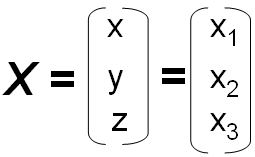Column of point coordinates or vector coefficients xa A component of the position vector r (or) Xkl Complex Fourier coefficient of the pixel at (k, l) yb A component of the position vector r (or) z Crystal thickness page4217 Z Average atomic number page4476 zc A component of the position vector r (or) r'; x', y', z'; or xi' Position vector and point coordinates after a transformation of a coordinate system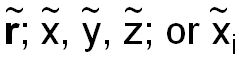New position vector and point coordinates after a symmetry operation u, v, w; or ui Integers, coordinates of a (primitive) lattice point; coefficients of vector u [uvw] Indices of a lattice direction or zone axis Indices of a set of all symmetrically equivalent lattice directions x, y, z; or xi Coordinates of a point (location of an atom) expressed in units of a, b, c; coordinates of end point of position vector r (or); coefficients of position vector r (or). ξ sense vector page3461 ξhkl Extinction distance page3769/page4134 φ(r) Electrostatic potential Φhkl Fourier coefficients of an electrostatic potential. page3880 ΦP Fourier transform of the projected electrostatic potential Ψexit Exit wave function from TEM Specimen page4217 ψillum Illumination wave function page3732 ψi(r) Image wave function page3732 ψo(r) Object wave function (in real space) page3732 ψo(u) Object wave function (in reciprocal space) page3732 Ψo,s Wave function of scattered electrons page4217 Φhkl Fourier coefficients of an electrostatic potential page3769 Φ1s(r) The lowest energy bound state of the atom column page4217 α A lattice or cell parameter: an interaxial (lattice) angle b ^ c α Electron beam convergence page3879 α* An interaxial (lattice) angle of reciprocal lattice b* ^ c* αmax Visibility band half-width α(hkl); or α Phase angle of the structure factor F(hkl) ρ Density of the material (g/cm3). page4475, page2673 ρ(xyz) Electron density at the point x,y,z β A lattice or cell parameter: an interaxial (lattice) angle c ^ a β Brightness of electron beam page4961 β Collection angle of EELS page3384 β2 Collection angle (mrad) page4476 β* An interaxial (lattice) angle of reciprocal lattice c* ^ a* γ A lattice or cell parameter: an interaxial (lattice) angle a ^ b γ* An interaxial (lattice) angle of reciprocal lattice a* ^ b* θ The scattering angle page3732 σ An interaction constant page4173 σ Mirror planes in crystals page1620 σ Cross section (of an incident electron interacting with an atom) page2673 σT Total scattering cross sectoin (including both elastic and inelastic scatterings, i.e. σelastic + σinelastic) for an isolated atom, page2673 Θ, or θ Half of the angle between the transmitted and scattered electron beams page3769 ϕ Phase λ Electron wavelength page4787 λ Mean free path (of an incident electron interacting with an atom) page2673 χ The phase shift from defocus and spherical aberration, phase-distortion function page3732 Δ Defocus spread page3879 Δ Difference parameter Δf Objective lens defocus Δdc Chromatic broadening page3384 ΔEL2,3 Separation of the L3 and L2 peak maxima page4770 ΔEL2,3 Chemical shift of Si L2,3 from that of pure silicon page3441 Δf Defocus page1715 δE Intrinsic energy spread of E0 page4165 Ω Solid angle subtended by the intersection of two visibility bands Γ Visibility factor in calculating lattice-fringe visibility band half-widths δ Dirac delta function τ A relativistic correction factor page4476into the page page4315 [ ] An orientation of a crystal [ ] A zone axis < > A group of orientations of a crystal ( ) A crystalline plane { } A group of planes of a crystal / Indicates parallel symmetry elements page2982 Schoenflies notation page1677

=================================================================================

The book author (Yougui Liao) welcomes your comments, suggestions, and corrections, please click here for submission. If you let book author know once you have cited this book, the brief information of your publication will appear on the “Times Cited” page.